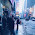## Saturday, 3 September 2016

### Formatting a date / time axis with specified value in v4

The following post is a section of the book 'D3 Tips and Tricks v4.x'.  The entire book can be downloaded in pdf format for free from Leanpub or you can read it online here.
Since this post is a snapshot in time. I recommend that you download a copy of the book which is updated frequently to improve and expand the content.
---------------------------------------
As referenced in the post where we initially developed our simple graph, the axes of that graph had no styling or configuration changes made to them at all. One of the results of this is that the font size, type, number of ticks and the way that the values are represented is very much at the default settings.

#### Formatting a date / time axis with specified values

OK then. We’ve been very clever in rotating our text, but you will notice that D3 has used its own good judgement as to what format the days / date will be represented as.
Not that there’s anything wrong with it, but what if we want to put a specific format of date / time nomenclature as axis labels?
No problem. D3 to the rescue again!
This is actually a pretty easy thing to do, but there are plenty of options for the formatting, so the only really tricky part is deciding what to put where.
But, before we start doing anything we are going to have to expand our bottom margin even more than we did with the rotate the axis labels feature.
That should see us right.
Now the simple part :-). Changing the format of the label is as simple as inserting the `tickFormat` command into the xAxis declaration and including a D3 time formatting function a little like this;
The `timeFormat` formatters are the same as those we used when parsing our time values when reading our data with the simple graph;
• %a - abbreviated weekday name.
• %A - full weekday name.
• %b - abbreviated month name.
• %B - full month name.
• %c - date and time, as “%a %b %e %H:%M:%S %Y”.
• %d - zero-padded day of the month as a decimal number [01,31].
• %e - space-padded day of the month as a decimal number [ 1,31].
• %H - hour (24-hour clock) as a decimal number [00,23].
• %I - hour (12-hour clock) as a decimal number [01,12].
• %j - day of the year as a decimal number [001,366].
• %m - month as a decimal number [01,12].
• %M - minute as a decimal number [00,59].
• %p - either AM or PM.
• %S - second as a decimal number [00,61].
• %U - week number of the year (Sunday as the first day of the week) as a decimal number [00,53].
• %w - weekday as a decimal number [0(Sunday),6].
• %W - week number of the year (Monday as the first day of the week) as a decimal number [00,53].
• %x - date, as “%m/%d/%y”.
• %X - time, as “%H:%M:%S”.
• %y - year without century as a decimal number [00,99].
• %Y - year with century as a decimal number.
• %Z - time zone offset, such as “-0700”.
• There is also a literal “%” character that can be presented by using double % signs.
So the format we have specified (`%Y-%m-%d`) will show the year with the century as a decimal number (`%Y`) followed by a hyphen, followed by a zero padded month as a decimal number (`%m`) followed by another hyphen and lastly the day as a zero padded day of the month (`%d`).
The end result looking a bit like this;Rotated x axis labels
An example using this code can be found on github or in the code samples bundled with this book (simple-axis-rotated-formatted.html and data.csv). A working example can be found on bl.ocks.org. The example code also includes the rotating of the x axis text as described in the previous section.
So how about we try something a little out of the ordinary (extreme)?
How about the full weekday name (`%A`), the day (`%d`), the full month name (`%B`) and the year (`%Y`) as a four digit number?
We will also need some extra space for the bottom margin, so how about 170?
And….Extreme format change for the x axis labels
Oh yeah… When axis ticks go bad…
But seriously, that does work as a pretty good example of the flexibility available.

The post above (and heaps of other stuff) is in the book 'D3 Tips and Tricks v4.x' that can be downloaded for free (or donate to encourage further development :-)).

#### 1 comment:

1.Hey, didn't find any way to contact you, but just wanted to write up this to thank you as this series of tutorials and examples helped me to go through and understand d3.js in under just two days :)

Used your example for gradient-filled areas and tweaked it around to "slope" the graph once data finishes to the end of the domain, giving a nicer look to graphs in which data is incomplete and does not reach out the full domain (i.e.: I have to show a full range of days in a graph, but I want all the remaining days that are not filled out to be sloped downwards to the end of the graph).

Worked around this by applying a quadratic polynomial which passes through a point A (end of graph data) to a vertex V (end of domain and interception of abscises at y=0). Demo can be found here: http://jsfiddle.net/rtroncoso/v94zd6g3/12/.

Feel free to look around, maybe it can help someone who's gone through the same problem and needs assistance with it. Keep it up with the good work, cheers!